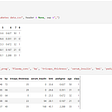# A Quick Tutorial to Encode List Variables

Practical data manipulation activities include categorical data that needs to be transformed in a way that a Machine Learning algorithm can understand. There are many ways of encoding this kind of data, some of which are Label Encoding or One-Hot Encoding. However, there are cases that categorical data encoding is not so straightforward, and additional data handling is necessary. One of those cases is when we have categorical data coming in a single column as a `list` , with each row belonging to several categories.

In this small article, I present a step-by-step tutorial on how to encode categorical data that comes as a `list` in a DataFrame column.

## Step 0: Generate some data

We are going to create a DataFrame from a list of dictionaries. As an example, let’s use some data holding the name of a person and the cities they have visited.

`import pandas as pddata = [    {'cities': ['Athens', 'London', 'Berlin'], 'person': 'John'},    {'cities': ['Athens', 'London'],  'person': 'Nick'},    {'cities': ['Berlin', 'London'],  'person': 'Helen'}]df = pd.DataFrame(data)`

The resulted DataFrame we constructed has a list of elements on each of the rows for the cities column.

We will go through a number of transformations that will give us a much easier-to-handle view of the same data, that will look like this:

## Step 1: Transform the column to a list

Next step is to transform the cities column to a list and put this into a DataFrame, which we’ll call `cities_df` .

`cities_df = pd.DataFrame(df['cities'].tolist())`

Note or Before applying Step 1. Sometimes, if we load the data from a file, we can’t be sure about the type of such a column. Depending on where the data comes from (e.g. derived from data crawling), the cities column might be type of `str` and therefore be loaded as type `str`; in this case, an additional preprocessing step is needed, before trying to apply the `tolist() `function to the column, to ensure that the input is evaluated correctly.
Using the `literal_eval()` function of the `ast` module, we can transform each element of the cities column. After that, we are ready to use the `tolist()` function on our data.

`import astdf['cities'] = df['cities'].apply(lambda x: ast.literal_eval(x))`

## Step 2: Transform columns to indexes

Then, we will need to transform our new DataFrame using `stack()` to stack our columns into indexes. This is a very useful function when it comes to rearranging our data and looking at it from a different perspective.

`cities_obj = cities_df.stack()`

and the result:

## Step 3: Convert to dummy variables

At this stage, we need to convert our categorical column into dummy variables. For this, we will use the pandas `get_dummies()` function, which is applied to each individual row in our object.

`cities_df = pd.get_dummies(cities_obj)`

## Step 4: Sum on the index level

We have started to approach the desired result. The goal is to sum the rows on the index level (level=0), in order to aggregate the information in one row for each case.

`cities_df = cities_df.sum(level=0)`

## Step 5: Re-join result with the original data

The resulting DataFrame is probably more meaningful if we re-join it with the original data that hold the rest of the information. We use concat() for this:

`cities_df = pd.concat([df, cities_df], axis=1)`

## Wrap-up

This article summarised the process of transforming this particular type of data. If you found this useful, apply it or like it :) otherwise, please leave feedback for alternative approaches!

--

--

--

## More from Christina Boididou

Data enthusiast, Machine Learning fan. Doing data work at the BBC

Love podcasts or audiobooks? Learn on the go with our new app.## Christina Boididou

Data enthusiast, Machine Learning fan. Doing data work at the BBC

## Setting up ML Environment with Anaconda## Handling Missing Data with Python## Project 1 - WomenTechWomenYes MTA TRAFFIC ANALYS